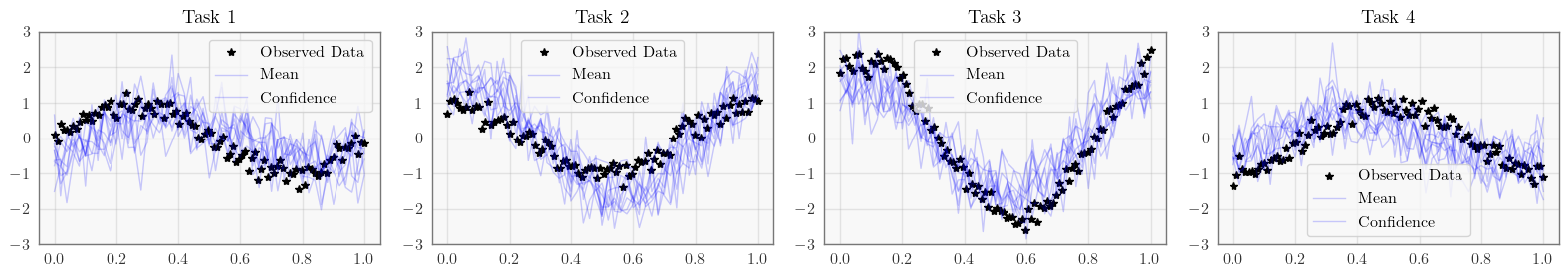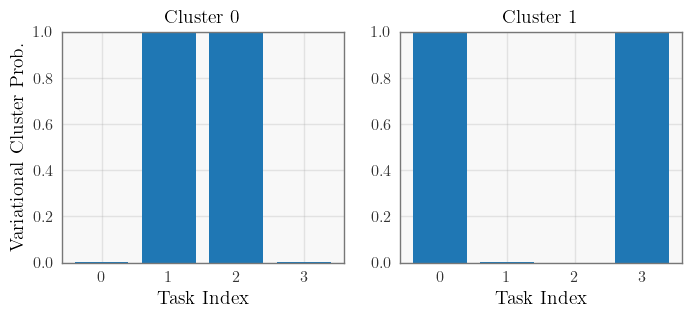# Clustered Multitask GP (w/ Pyro/GPyTorch High-Level Interface)¶

## Introduction¶

In this example, we use the Pyro integration for a GP model with additional latent variables.

We are modelling a multitask GP in this example. Rather than assuming a linear correlation among the different tasks, we assume that there is cluster structure for the different tasks. Let’s assume there are $$k$$ different clusters of tasks. The generative model for task $$i$$ is:

$p(\mathbf y_i \mid \mathbf x_i) = \int \sum_{z_i=1}^k p(\mathbf y_i \mid \mathbf f (\mathbf x_i), z_i) \: p(z_i) \: p(\mathbf f (\mathbf x_i) ) \: d \mathbf f$

where $$z_i$$ is the cluster assignment for task $$i$$. There are therefore $$k$$ latent functions $$\mathbf f = [f_1 \ldots f_k]$$, each modelled by a GP, representing each cluster.

Our goal is therefore to infer:

• The latent functions $$f_1 \ldots f_k$$

• The cluster assignments $$z_i$$ for each task

:

import math
import torch
import pyro
import tqdm
import gpytorch
from matplotlib import pyplot as plt

%matplotlib inline
import matplotlib as mpl
mpl.rc_file_defaults()

# this is for running the notebook in our testing framework
import os
smoke_test = ('CI' in os.environ)


The standard GPyTorch variational objects will take care of inferring the latent functions $$f_1 \ldots f_k$$. However, we do need to add the additional latent variables $$z_i$$ to the models. We will do so by creating a custom likelihood that models:

$\sum_{z_i=1}^k p(\mathbf y_i \mid \mathbf f (\mathbf x_i), z_i) \: p(z_i)$

GPyTorch’s likelihoods are capable of modeling additional latent variables. Our custom likelihood needs to define the following three functions:

• pyro_model (needs to call through to super().pyro_model at the end), which defines the prior distribution for additional latent variables

• pyro_guide (needs to call through to super().pyro_guide at the end), which defines the variational (guide) distribution for additional latent variables

• forward, which defines the observation distributions conditioned on \mathbf f (\mathbf x_i) and any additional latent variables.

### The pyro_model function¶

For each task, we will model the cluster assignment with a OneHotCategorical variable, where each cluster has equal probability. The pyro_model function will make a pyro.sample call to this prior distribution and then call the super method:

# self.prior_cluster_logits = torch.zeros(num_tasks, num_clusters)

def pyro_model(self, function_dist, target):
cluster_assignment_samples = pyro.sample(
self.name_prefix + ".cluster_logits",  # self.name_prefix is added by PyroGP
pyro.distributions.OneHotCategorical(logits=self.prior_cluster_logits).to_event(1)
)
return super().pyro_model(
function_dist,
target,
cluster_assignment_samples=cluster_assignment_samples
)


Note that we are adding an additional argument cluster_assignment_samples to the super().pyro_model call. This will pass the cluster assignment samples to the forward call, which is necessary for inference.

### The pyro_guide function¶

For each task, the variational (guide) diustribution will also be a OneHotCategorical variable, which will be defined by the parameter self.variational_cluster_logits. The pyro_guide function will make a pyro.sample call to this prior distribution and then call the super method:

def pyro_guide(self, function_dist, target):
pyro.sample(
self.name_prefix + ".cluster_logits",  # self.name_prefix is added by PyroGP
pyro.distributions.OneHotCategorical(logits=self.variational_cluster_logits).to_event(1)
)
return super().pyro_guide(function_dist, target)


Note that we are adding an additional argument cluster_assignment_samples to the super().pyro_model call. This will pass the cluster assignment samples to the forward call, which is necessary for inference.

### The forward function¶

The pyro_model fuction passes the additional keyword argument cluster_assignment_samples to the forward call. Therefore, our forward method will define the conditional probability $$p(\mathbf y_i \mid \mathbf f(\mathbf x), z_i)$$, where $$\mathbf f(\mathbf x)$$ corresponds to the variable function_samples and $$z_i$$ corresponds to the variable cluster_assignment_samples.

In our example $$p(\mathbf y_i \mid \mathbf f(\mathbf x), z_i)$$ corresponds to a Gaussian noise model.

# self.raw_noise is the Gaussian noise parameter
# function_samples is n x k
# cluster_assignment_samples is k x t, where t is the number of tasks

def forward(self, function_samples, cluster_assignment_samples):
return pyro.distributions.Normal(
loc=(function_samples.unsqueeze(-2) * cluster_assignment_samples).sum(-1),
scale=torch.nn.functional.softplus(self.raw_noise).sqrt()
).to_event(1)
# The to_event call is necessary because we are returning a multitask distribution,
# where each task dimension corresponds to each of the t tasks


This is all we need for inference! However, if we want to use this model to make predictions, the cluster_assignment_samples keyword argument will not be passed into the function. Therefore, we need to make sure that forward can handle both inference and predictions:

def forward(self, function_samples, cluster_assignment_samples=None):
if cluster_assignment_samples is None:
# We'll get here at prediction time
# We'll use the variational distribution when making predictions
cluster_assignment_samples = pyro.sample(
self.name_prefix + ".cluster_logits", self._cluster_dist(self.variational_cluster_logits)
)

return pyro.distributions.Normal(
loc=(function_samples.unsqueeze(-2) * cluster_assignment_samples).sum(-1),
scale=torch.nn.functional.softplus(self.raw_noise).sqrt()
).to_event(1)

:

class ClusterGaussianLikelihood(gpytorch.likelihoods.Likelihood):
super().__init__()

# These are parameters/buffers for the cluster assignment latent variables

# The Gaussian observational noise
self.register_parameter("raw_noise", torch.nn.Parameter(torch.tensor(0.0)))

# Other info
self.num_clusters = num_clusters
self.max_plate_nesting = 1

def pyro_guide(self, function_dist, target):
# Here we add the extra variational distribution for the cluster latent variable
pyro.sample(
self.name_prefix + ".cluster_logits",  # self.name_prefix is added by PyroGP
pyro.distributions.OneHotCategorical(logits=self.variational_cluster_logits).to_event(1)
)
return super().pyro_guide(function_dist, target)

def pyro_model(self, function_dist, target):
# Here we add the extra prior distribution for the cluster latent variable
cluster_assignment_samples = pyro.sample(
self.name_prefix + ".cluster_logits",  # self.name_prefix is added by PyroGP
pyro.distributions.OneHotCategorical(logits=self.prior_cluster_logits).to_event(1)
)
return super().pyro_model(function_dist, target, cluster_assignment_samples=cluster_assignment_samples)

def forward(self, function_samples, cluster_assignment_samples=None):
# For inference, cluster_assignment_samples will be passed in
# This bit of code is for when we use the likelihood in the predictive mode
if cluster_assignment_samples is None:
cluster_assignment_samples = pyro.sample(
self.name_prefix + ".cluster_logits",
pyro.distributions.OneHotCategorical(logits=self.variational_cluster_logits).to_event(1)
)

# Now we return the observational distribution, based on the function_samples and cluster_assignment_samples
res = pyro.distributions.Normal(
loc=(function_samples.unsqueeze(-2) * cluster_assignment_samples).sum(-1),
scale=torch.nn.functional.softplus(self.raw_noise).sqrt()
).to_event(1)
return res


## Constructing the PyroGP model¶

The PyroGP model is essentially the same as the model we used in the simple example, except for two changes

• We now will use our more complicated ClusterGaussianLikelihood

• The latent function should be vector valued to correspond to the k latent functions. As a result, we will learn a batched variational distribution, and use a IndependentMultitaskVariationalStrategy to convert the batched variational distribution into a MultitaskMultivariateNormal distribution.

:

class ClusterMultitaskGPModel(gpytorch.models.pyro.PyroGP):
def __init__(self, train_x, train_y, num_functions=2, reparam=False):
num_data = train_y.size(-2)

# Define all the variational stuff
inducing_points = torch.linspace(0, 1, 64).unsqueeze(-1)
variational_distribution = gpytorch.variational.CholeskyVariationalDistribution(
num_inducing_points=inducing_points.size(-2),
batch_shape=torch.Size([num_functions])
)

# Here we're using a IndependentMultitaskVariationalStrategy - so that the output of the
# GP latent function is a MultitaskMultivariateNormal
gpytorch.variational.VariationalStrategy(self, inducing_points, variational_distribution),
)

# Standard initializtation
likelihood = ClusterGaussianLikelihood(train_y.size(-1), num_functions)
super().__init__(variational_strategy, likelihood, num_data=num_data)
self.likelihood = likelihood
self.num_functions = num_functions

# Mean, covar
self.mean_module = gpytorch.means.ZeroMean()
self.covar_module = gpytorch.kernels.ScaleKernel(gpytorch.kernels.RBFKernel())

def forward(self, x):
mean_x = self.mean_module(x)
covar_x = self.covar_module(x)
res = gpytorch.distributions.MultivariateNormal(mean_x, covar_x)
return res


This model can now be used to perform inference on cluster assignments, as well as make predictions using the inferred cluster assignments!

:

train_x = torch.linspace(0, 1, 100)
train_y = torch.stack([
torch.sin(train_x * (2 * math.pi)) + torch.randn(train_x.size()) * 0.2,
torch.cos(train_x * (2 * math.pi)) + torch.randn(train_x.size()) * 0.2,
torch.sin(train_x * (2 * math.pi)) + 2 * torch.cos(train_x * (2 * math.pi)) + torch.randn(train_x.size()) * 0.2,
-torch.cos(train_x * (2 * math.pi)) + torch.randn(train_x.size()) * 0.2,
], -1)

:

pyro.clear_param_store()
num_particles = 1 if smoke_test else 64
num_iter = 4 if smoke_test else 1000

def train(lr=0.01):
elbo = pyro.infer.Trace_ELBO(num_particles=num_particles, vectorize_particles=True, retain_graph=True)
svi = pyro.infer.SVI(model.model, model.guide, optimizer, elbo)
model.train()

iterator = tqdm.notebook.tqdm(range(num_iter))
for i in iterator:
loss = svi.step(train_x, train_y)
iterator.set_postfix(loss=loss)

%time train()


CPU times: user 1min 29s, sys: 2.59 s, total: 1min 32s
Wall time: 15.4 s

:

# Set into eval mode and make predictions
model.eval()
test_x = torch.linspace(0, 1, 51)
predictions = model.likelihood(model(test_x)).rsample()
print(predictions.shape)  # num_samples x num_data x num_tasks

ax.plot(test_x.numpy(), predictions[:, :, task].T.numpy(), 'b', lw=1, alpha=0.2)
ax.set_ylim([-3, 3])
ax.legend(['Observed Data', 'Mean', 'Confidence'])

fig.tight_layout()
None

torch.Size([10, 51, 4]):

# Infer cluster assignments
variational_clusters = pyro.distributions.Categorical(logits=model.likelihood.variational_cluster_logits).probs

# Plot cluster assignments
fig, axs = plt.subplots(1, model.num_functions, figsize=(4 * model.num_functions, 3))
for function, ax in enumerate(axs):[ ]: